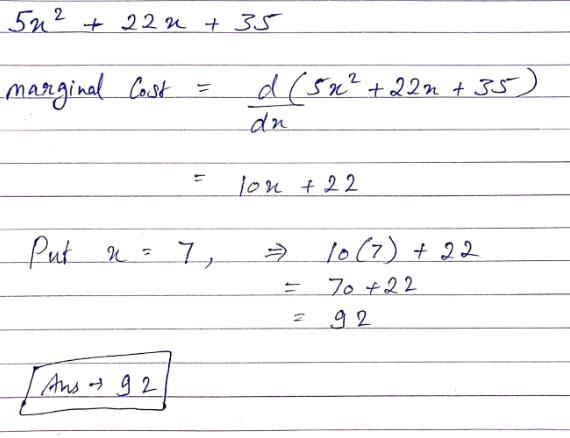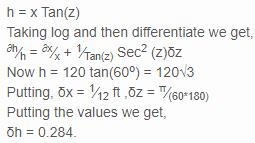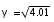# Test: Rate Of Change And Approximations

## 10 Questions MCQ Test Mathematics (Maths) Class 12 | Test: Rate Of Change And Approximations

Description
This mock test of Test: Rate Of Change And Approximations for JEE helps you for every JEE entrance exam. This contains 10 Multiple Choice Questions for JEE Test: Rate Of Change And Approximations (mcq) to study with solutions a complete question bank. The solved questions answers in this Test: Rate Of Change And Approximations quiz give you a good mix of easy questions and tough questions. JEE students definitely take this Test: Rate Of Change And Approximations exercise for a better result in the exam. You can find other Test: Rate Of Change And Approximations extra questions, long questions & short questions for JEE on EduRev as well by searching above.
QUESTION: 1

Solution:
QUESTION: 2

### The total revenue in Rupees received from the sale of x units of a product is given by R(x) = 5x2 + 22x + 35. Find the marginal revenue, when x = 7, where by marginal revenue we mean the rate of change of total revenue with respect to the number of items sold at an instant

Solution:QUESTION: 3

### Find the approximate change in total surface area of a cube of side x metre caused by increase in side by 1%.​

Solution:
QUESTION: 4

The volume of cube is increasing at the constant rate of 3 cm3/s. Find the rate of change of edge of the cube when its edge is 5 cm.​

Solution:

Let V be the instantaneous volume of the cube.
dV/dt = 3 cm3/s
Let x be the side of the cube.
V = x3
dV/dt = 3x2 * (dx/dt)
3 = 3*(52)*(dx/dt)
So, dx/dt = 1/25 cm/s

QUESTION: 5

The total cost associated with the production of x units of a product is given by C(x) = 5x2 + 14x + 6. Find marginal cost when 5 units are produced

Solution:

C(x) = 5x2 + 14x + 6
Marginal Cost M(x) = C’(x)
M(x) = 10x +14
So, M(5) = 50 + 14
= 64 Rs

QUESTION: 6

At a distance of 120ft from the foot of a tower the elevation of its top is 60o. If the possible error in measuring the distance and elevation are 1 inch and 1 minute, find approximate error in calculated height.

Solution:

Tan(z) = hxQUESTION: 7

A stone is dropped into a quiet lake and waves move in circles at a speed of 2cm per second. At the instant, when the radius of the circular wave is 12 cm, how fast is the enclosed area changing ?​

Solution:

Rate of increase of radius dr/dt = 2 cm/s
Area of circle A = πr2
dA/dt = π*(2r)*(dr/dt)
= π*(24)*2
= 48π cm2/s
Rate of increase of area is 48π cm2/s (increasing as it is positive).

QUESTION: 8

Using approximation find the value ofSolution:

Let x=4, Δx=0.01

y=x^½ = 2

y+Δy = (x+ Δx)^½ = (4.01)^½

Δy = (dy/dx) * Δx

Δy = (x^(-1/2))/2 * Δx

Δy = (½)*(½) * 0.01

Δy = 0.25 * 0.01

Δy = 0.0025

So, (4.01)^½ = 2 + 0.0025 = 2.0025

QUESTION: 9

Find the approximate value of f(10.01) where f(x) = 5x2 +6x + 3​

Solution:

f(x) = 5x2 +6x + 3
f(10.01) = 5*(10.01)2 + 6*(10.01) + 3
To find (10.01)2
Let p=10, Δp=0.01
y=p2 = 100
y+Δy = (p+ Δp)2 = (10.01)2
Δy = (dy/dp) * Δp
Δy = 2*p* Δx
Δy = 2*10* 0.01
Δy = 20 * 0.01
Δy = 0.2
So, (10.01)2 = y + Δy
= 100.2
So,
f(10.01) = 5*(100.2) + 6*(10.01) + 3
= 501 + 60.06 + 3
= 564.06

QUESTION: 10

Given a function y = f(x) . Let Δx be the very small change in the value of x , then the corresponding change in the value of y that is Δy is approximately given by

Solution: